### Home > MC2 > Chapter 8 > Lesson 8.2.4 > Problem8-70

8-70.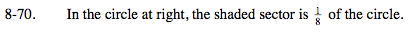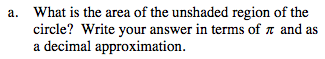If the unshaded region were half of the circle, then its area would be half that of the entire circle.
Begin by determining what portion of the circle is unshaded and by finding the circle's total area.

Area of a circle = π · r2 = 25?

$\text{If }\frac{1}{8}\text{ of the circle is shaded, what fraction is unshaded?}$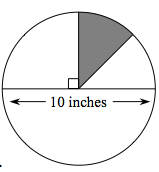$\frac{175}{8} \pi \approx$

68.72 square inches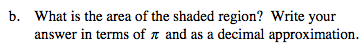See part (a).

$\text{What is }\frac{1}{8}\text{ of the total area of the circle (25}\pi)?$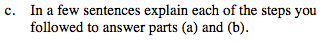What strategy did you use to solve for parts (a) and (b)?
Describe how you were able to find the area of the various portions of the circle.